# Lesson 6-I: Interest-Bearing Accounts and Finite Difference Models

Note: This lesson and Lesson 6-II are really two parts of one whole. It's advisable to either do them at the same time, or to do Lesson 6-II very shortly after completing Lesson 6-I.

This lesson deals with the time value of money and compound interest. We will examine interest-bearing savings accounts with regular monthly deposits (every month we deposit the same amount). How long will it take to save a target amount? If we want a target amount by some deadline, how much do we need to save each month?

# The Mathematical Model

In an interest-bearing account, the amount you have currently (we'll say "this month") is: the prior amount (balance "last month"), plus the interest you earned (say that month), plus any deposits you made that month. As a word equation: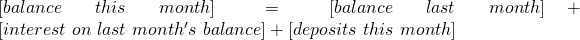The prior balance will have a formula like this one, and we'll keep track of how much we're depositing, so only the middle term needs to be fleshed out:where we divided by 12 because interest rates are reported on an annual basis and we're working on a monthly basis.

Let's make our word equations symbolic.

Clearly time is an independent variable in this situation. We'll work on the standard legal and business assumption (which happens to be completely false) that all months have the same length, and we'll use that length as our unit of time measurement. The other variables and parameters in play are:

 variable/parameter interpretation unitstime monthsbalance $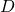monthly deposit$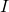annual interest rate

We've left the units ofblank because percentages don't have units. The notationindicates that we're treating this problem as discrete, that is, we're just checking our account balance once a month, rather than knowing the balance at all times.  Using these symbols, we can translate our word equation into a symbolic equation: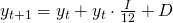which simplifies to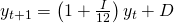This is known as a finite difference model, because it tells us what the difference between the values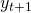and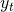is, and the step size is one month, which is a positive number (so its reciprocal,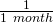is finite).

# The Iterative Method

There are two methods to compute the account balance in a given month from a finite difference model. One is using iteration (which is just a fancy way to say "do the same arithmetic over and over"); the other is to use algebra (which is just a fancy way to say "do some arithmetic once and for all"). We'll (make the computer) do arithmetic here, and then (make the computer) do algebra in the next lesson.

Excel is very good for tedious arithmetic, so we'll use it to showcase the iterative method. Let's get some numbers.

We'll assume the annual interest rate is 3%, we have an initial deposit of $3000, and we can put away$150 each month. We'd like to know the answer to questions like:

1. How will take us to save up $10,000? 2. If we want to save$10,000 in 24 months, what does our monthly deposit have to be?

a video walkthrough of the iterative method in Excel

First open up an Excel sheet. In column A, place the label "t" for which month it is, and the fill column A with months, starting from 0 (when we open the account) and increasing by 1 each row. In column B, put a label "y" and enter "3000" in cell B2 because our initial deposit will be $3000.Somewhere off to the side, say in E1 and F1, enter "I" and "D" as labels for our parameters. Below them, enter the values "0.03" and "150" respectively. In cell B3, enter the formula "=(1+E$2/12)*B2+F$2"; this is just the Excelified version of our finite-difference model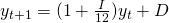. Note the$ in the formula, which will allow us to copy-and-paste the formula.The word iteration comes from the Latin word iter, which means "again": so let's do it again. Copy and paste the formula in cell B3 into cells B4 through B12. You should get something like:which shows that, at the end of 10 months we have nearly $4,600 in the account. To see how long it takes to get$10,000 in the account, we could keep copying-and-pasting; or using Excel's pattern-recognition skills, highlight cells A3:B12 and drag down on the lower-right corner.

Either way, it'll be a while till you get $10,000 in column B (after 24 months we still only have$6890.69).

What we just did took an instant, but imagine the work involve to do it by hand: many divisions, multiplications, additions. And if you made a mistake in computing the balance in month 15, say, then that mistake would have propagated through all subsequent months. That's why banks and firms used to employ teams of clerical workers whose sole job was to do arithmetic (and, sometimes, more complicated computations). These workers were known as "computers", and the job was more often than not veiwed as women's work because it was so unglamorous. Nevertheless, getting those computations right was what powered business big and small before the invention of electronic adding machines.

So much for question (1). To answer question (2), we could guess and check various values of D in cell F2 to see which gets us $10,000 in cell B26 (we wouldn't want to get to$10,000 before then -- we need some walking around money so we don't want to save too much). Guess-and-check isn't a very elegant solution, but it works.

# Takeaways/Deliverables

To say you've successfully completed this lesson, you should be able to do the following:

1. Derive a finite difference model for compound interest, given rate and regular monthly deposit.
2. Use an Excel spreadsheet to carry out the iterative method of computing compound interest.
3. Use the iterative method to find the required regular deposit required to obtain a desired account balance in a desired time period.

These skills are what you'll need to complete the WebAssign homework for this Lesson.